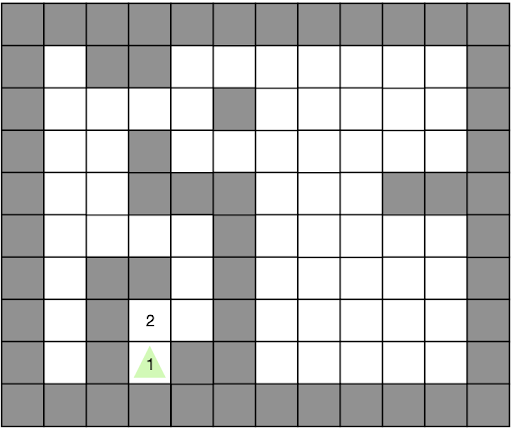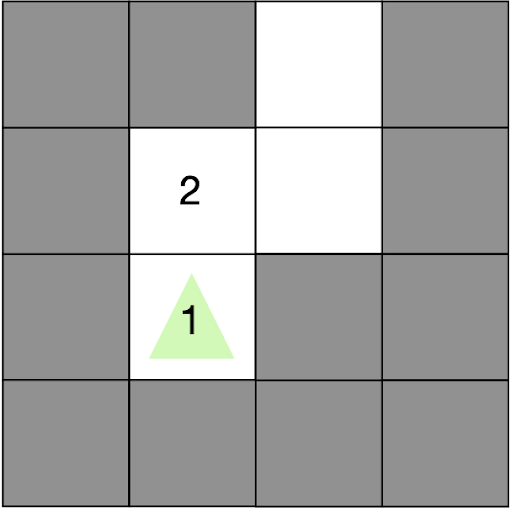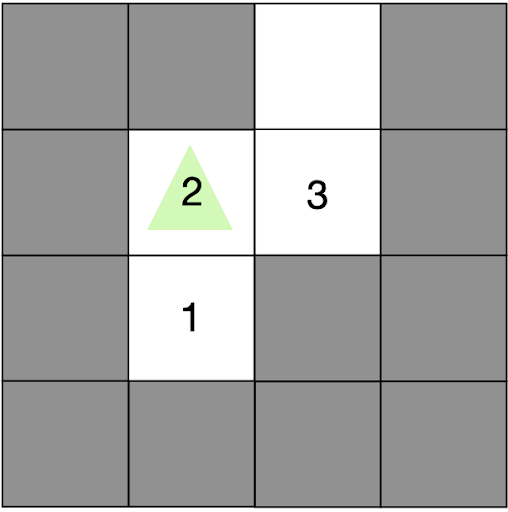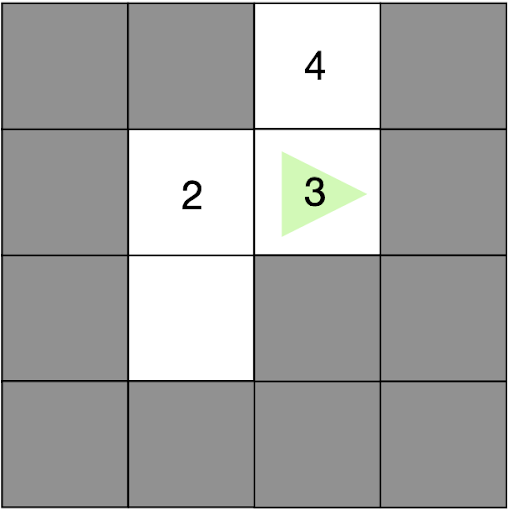# Lesson 3: Conditionals with HotRider### Introduction

Hi! My name is HotRider. We will find hidden treasure on the map by playing the game Hot and Cold. Treasure is hidden on some of the tiles. My sensor values show how “hot” the surrounding tiles are. Help me reach the treasure by moving in the right direction.

### Conditional Expressions

In this lesson, we will learn to use conditional expressions in robotics coding. We need to use conditional statements to let the robot decide what action it will take based on whether a statement is true or false. For example, if it is true that the tile in front of us is ‘hotter’, then we may decide to move forward. If it is true that the tile to the right is ‘hotter’, then we we can turn right and move forward. A ‘hotter’ tile means the tile has a number bigger than the number we are currently on.

### Getting Started

1. Start the simulation and open the simulation screen.
2. You will see the number 2 on the tile in front of the robot, as shown in the image below. The robot is on top of a tile with number 1. Since 2 is higher than 1, this tells the robot that moving forward will take us from 1 to 2. Moving to higher numbers means we are getting closer to the treasure.
3. The walls have been given a value of -1000. We can read this with our sensors but we do not show wall values to avoid clutter.
4. After the robot moves you will be able to see the sensor values of the new position.### Robot Commands

1. robot.move_forward() – Moves the robot 1 tile forward.
2. robot.turn_right() – Rotates the robot 90° clockwise.
3. robot.turn_left() – Rotates the robot 90° counter clockwise.
4. robot.move_backward() – Moves the robot 1 tile backward.
5. robot.is_ok() – True when the simulation is running.
6. robot.front, robot.center, robot.left, robot.right – These variables give you the current sensor values of the neighboring tiles.

### Checkpoints

Checkpoint #1 – Your first conditional

1. Before starting to code, write simple print commands to see the values of robot.center, robot.front, robot.left and robot.right variables on the terminal and run your code. To access the terminal, right click on Node: Test Node under NODES and select Watch Node Logs.

The image below shows the situation you start in. If the tile in front is ‘hotter’ we need to move forward.Try adding the following conditional to do this:
while robot.is_ok():

if robot.front == robot.center + 1:

robot.move_forward()

1.
• Run the simulation and watch the motion of the robot.

Checkpoint #2 – Adding a conditional for the right turn

• The image below shows the new position for the robot. If the tile to the right is ‘hotter’ we need to turn right.• Try adding another conditional, similar to the first, so the robot can turn right.
• Run the simulation and watch the motion of the robot.

Checkpoint #3 – Adding the left turn and finding all the treasure

• The image below shows the new position for the robot. If the tile to the left is ‘hotter’ we need to turn left.• Try adding another conditional, similar to the first two, so the robot can turn left.
• Run the simulation and watch the motion of the robot.
• Now you should see the robot find all three treasure points and complete the course. You may have noticed that our robot is making extra right and left rotation movements while going to the final destination? Or perhaps you already solved this problem. Let’s look at how the robot can reach the target with less energy.

Checkpoint #4 – Using ‘elif‘ to reach the target with less energy.

Intead of using if commands, we can use elif (else if) so that only one conditional can execute on each loop. Look at the example code below. Both step1() and step2() will happen if condition2 is true after step1().

if condition1:

step1()

if condition2:

step2()

Now consider the same code but with elif. In this case step2() will not be called if step1() was called.

if condition1:

step1()

elif condition2:

step2()

•
Try changing your code to use elif. Does this resolve the extra turning?

### Challenge

• Try to write an algorithm which completes the course using only the data from the front sensor.
• Hint: You will have to make extra turns to search for the correct path.